RRB Group D Mock Test - 37

# RRB Group D Mock Test - 37

Test Description

## 100 Questions MCQ Test RRB (Group D) - Mock Tests & Previous Year Papers | RRB Group D Mock Test - 37

RRB Group D Mock Test - 37 for Railways 2023 is part of RRB (Group D) - Mock Tests & Previous Year Papers preparation. The RRB Group D Mock Test - 37 questions and answers have been prepared according to the Railways exam syllabus.The RRB Group D Mock Test - 37 MCQs are made for Railways 2023 Exam. Find important definitions, questions, notes, meanings, examples, exercises, MCQs and online tests for RRB Group D Mock Test - 37 below.
Solutions of RRB Group D Mock Test - 37 questions in English are available as part of our RRB (Group D) - Mock Tests & Previous Year Papers for Railways & RRB Group D Mock Test - 37 solutions in Hindi for RRB (Group D) - Mock Tests & Previous Year Papers course. Download more important topics, notes, lectures and mock test series for Railways Exam by signing up for free. Attempt RRB Group D Mock Test - 37 | 100 questions in 90 minutes | Mock test for Railways preparation | Free important questions MCQ to study RRB (Group D) - Mock Tests & Previous Year Papers for Railways Exam | Download free PDF with solutions
 1 Crore+ students have signed up on EduRev. Have you?
RRB Group D Mock Test - 37 - Question 1

### A shopkeeper sold an item at a loss of 20% and received 20000/-. What was the cost price of the item?

Detailed Solution for RRB Group D Mock Test - 37 - Question 1

Let CP = x

(1-0.2)(x) = 20000

(0.8)(x) = 20000

x = 25000/-

So the answer is option B.

RRB Group D Mock Test - 37 - Question 2

### A college celebrated a festival for five days. The average daily attendance in those five days was 678 while the average attendance in the last four days of the festival was 679. What was the attendance on the first day of the festival?

Detailed Solution for RRB Group D Mock Test - 37 - Question 2

Total attendance in 5 days = 678*5 = 3390

Attendance in 1st day + attendance in last 4 days = attendance in 5 days

x + 4(679) = 3390

x + 2716 = 3390

x = 674

So the answer is option D.

RRB Group D Mock Test - 37 - Question 3

### Rupesh started his journey of 150km with the speed of 45kmph, after travelling for 2hrs, he realized that he is left with 1hr to reach his destination. Find his new speed if he reached his destination on-time.

Detailed Solution for RRB Group D Mock Test - 37 - Question 3

Total distance = 150km

Distance covered by him in 2 hrs = 45*2 = 90km

Remaining distance = 150-90 = 60km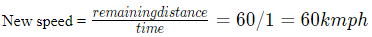So the answer is option C.

RRB Group D Mock Test - 37 - Question 4

Find tan θ, if secθ = 13/12 ?
It is known that 0 < θ < 90

Detailed Solution for RRB Group D Mock Test - 37 - Question 4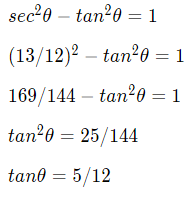So the answer is option B.

RRB Group D Mock Test - 37 - Question 5

Find the HCF of 1/2, 2/3 & 3/4 ?

Detailed Solution for RRB Group D Mock Test - 37 - Question 5

H.C.F of fractions = H.C.F of numerators / L.C.M of denominators = (HCF of 1, 2, 3)/(LCM of 2, 3, 4) = (1)/(12) = 1/12

RRB Group D Mock Test - 37 - Question 6

P and Q together can complete a piece of work in 8 days, Q alone can complete the same work in 12 days.In how many days can P alone complete the same work?

Detailed Solution for RRB Group D Mock Test - 37 - Question 6

Let total work = LCM of 8 and 12 = 24 units

Efficiency of P&Q; = 24/8 = 3

Efficiency of Q = 24/12 = 2

Then efficiency of P = 3-2 = 1

P alone can complete 24 units of work in 24/1 = 24 days

So the answer is option A.

RRB Group D Mock Test - 37 - Question 7

If a+b+c=0, then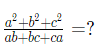Detailed Solution for RRB Group D Mock Test - 37 - Question 7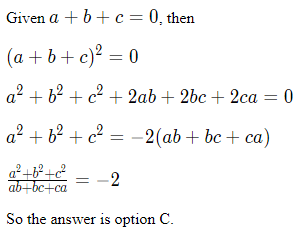RRB Group D Mock Test - 37 - Question 8

Find the side of an equilateral triangle, of area 25√3​ sq.cm ?

Detailed Solution for RRB Group D Mock Test - 37 - Question 8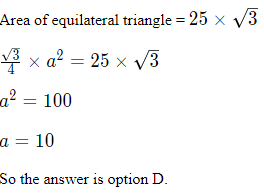RRB Group D Mock Test - 37 - Question 9

Find a-b if a+b = 23, ab = 132 ?

Detailed Solution for RRB Group D Mock Test - 37 - Question 9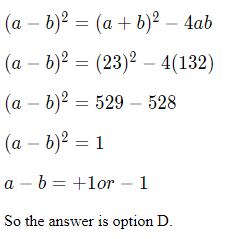RRB Group D Mock Test - 37 - Question 10

Find the perimeter of a rectangle whose length is 9 cm and breadth is equal to the side of a square of area 36 sq.cm ?

Detailed Solution for RRB Group D Mock Test - 37 - Question 10

Length of rectangle = 9 cm

Breadth = side of square = √36 = 6 cm

Perimeter = 2(l+b) = 2(9+6) = 30cm

So the answer is option B.

RRB Group D Mock Test - 37 - Question 11

4 walls of a house of height 4m, width 6m and length 8m are to be painted. What is the total expenditure if the painter charges Rs.1.5 per sq.m ?

Detailed Solution for RRB Group D Mock Test - 37 - Question 11

Lateral surface area = 2h(l+b) = 2(4)(6+8) = 112sq.cm

Cost of painting = 112*1.5 = 168

So the answer is option A.

RRB Group D Mock Test - 37 - Question 12

Sampath sold an item to sharath for 2500/- and earned a profit of 100/-. What is the profit percentage in this transaction?

Detailed Solution for RRB Group D Mock Test - 37 - Question 12

SP = 2500

Profit = 100

CP = SP - Profit = 2500 - 100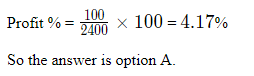RRB Group D Mock Test - 37 - Question 13

If sum of a number and 4 times its fraction is 4, then find that number ?

Detailed Solution for RRB Group D Mock Test - 37 - Question 13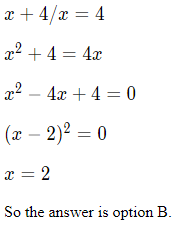RRB Group D Mock Test - 37 - Question 14

Find the slope of a line perpendicular to 5x-3y+2 = 0 ?

Detailed Solution for RRB Group D Mock Test - 37 - Question 14

Slope of given line = 5/3

Slope of a line perpendicular to given line = -3/5

So the answer is option C.

RRB Group D Mock Test - 37 - Question 15

p and q are the total surface area and curved surface area of a cylinder. If the height and radius of the cylinder are the same, what is the value of p/q ?

Detailed Solution for RRB Group D Mock Test - 37 - Question 15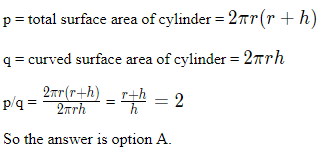RRB Group D Mock Test - 37 - Question 16

The edges of a cuboid are in the ratio 2 : 3 : 4. If the total surface area of the cuboid is 468 sq cm, what is its volume?

Detailed Solution for RRB Group D Mock Test - 37 - Question 16

Let it's edges are 2x, 3x, 4x

Surface area = 468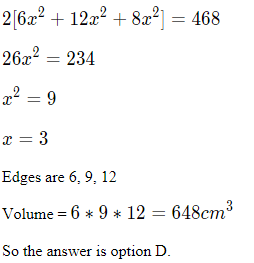RRB Group D Mock Test - 37 - Question 17

If p, qp,q are the sum and product of the roots of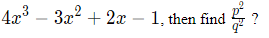Detailed Solution for RRB Group D Mock Test - 37 - Question 17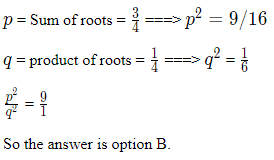RRB Group D Mock Test - 37 - Question 18

A circle is inscribed in a square of side 14cm, then find the maximum area of the circle ?

Detailed Solution for RRB Group D Mock Test - 37 - Question 18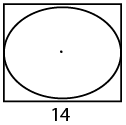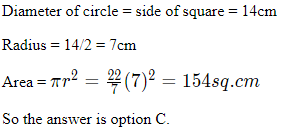RRB Group D Mock Test - 37 - Question 19

Train P of length 300m and Train Q of length 600m are travelling with 48kmph and 66kmph respectively. Find the time taken by them to cross each other if they are moving in same direction ?

Detailed Solution for RRB Group D Mock Test - 37 - Question 19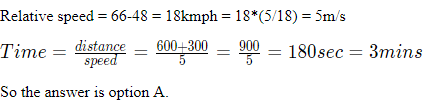RRB Group D Mock Test - 37 - Question 20

Find the area of trapezium ABCD, AB=7 cm, CD=5 cm & height of trapezium is 4 cm ? It is known that AB is parallel to CD

Detailed Solution for RRB Group D Mock Test - 37 - Question 20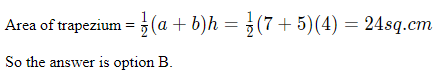RRB Group D Mock Test - 37 - Question 21

Select the option that bears the same relationship as the terms given in the question:
BA : CE :: DI : ?

Detailed Solution for RRB Group D Mock Test - 37 - Question 21

B is the first consonant and A is the first vowel.
C is the second consonant and E is the second vowel.
D is the third consonant and I is the third vowel.

Therefore, the next term must be composed of the fourth consonant and the fourth vowel - F and O respectively. Therefore, option C is the right answer.

RRB Group D Mock Test - 37 - Question 22

elect the option that is different from the other 3 options:

Detailed Solution for RRB Group D Mock Test - 37 - Question 22

Kiwi, Ostrich and Emu are flightless birds. Peacock can fly. Therefore, option D is the right answer.

RRB Group D Mock Test - 37 - Question 23

Find the order in which the following words appear in a dictionary.
1. nemesis
2. neology
3. nefarious
4. neonatology

Detailed Solution for RRB Group D Mock Test - 37 - Question 23

The order in which these words will appear in a dictionary:
3. nefarious
1. nemesis
2. neology
4. neonatology

RRB Group D Mock Test - 37 - Question 24

Find the number which will replace ‘?’.
4, 18, 48, 100, ?

Detailed Solution for RRB Group D Mock Test - 37 - Question 24

The series follows the following pattern n3−n2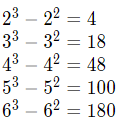RRB Group D Mock Test - 37 - Question 25

Which of the following words cannot be formed using the letters of the word ‘SCHADENFREUDE’?

Detailed Solution for RRB Group D Mock Test - 37 - Question 25

Diffuser has 2 F’s. SCHADENFREUDE has only one F. Clearly, DIFFUSER cannot be made by shuffling the letters of SCHADENFREUDE. Hence, option D is the right answer.

RRB Group D Mock Test - 37 - Question 26

If DRY is coded as 1800 and BAT is coded as 40, the code of which of the following words is 200?

Detailed Solution for RRB Group D Mock Test - 37 - Question 26

The code for a word is the product of the position of the letters of the word in the alphabetical series.

DRY - 4*18*25 = 1800
BAT - 2*1*20 = 40

Let us evaluate the options using the same logic.

GATE - 7*1*20*5 = 700
DEBT - 4*5*2*20 - 800
BEED - 2*5*5*4 - 200
DATE - 4*1*20*5 - 400
Therefore, option C is the right answer.

RRB Group D Mock Test - 37 - Question 27

If ‘+’ means ‘-’, ‘-’ means ‘*’, ‘*’ means ‘/’ and ‘/’ means ‘+’, then what is the value of the following expression?
13 - 13 / 3 - 63 * 9 + 2 -5

Detailed Solution for RRB Group D Mock Test - 37 - Question 27

Let us replace the symbols with the actual operators.

13 - 13 / 3 - 63 * 9 + 2 -5 = 13 * 13 + 3*63/9 - 2*5

Applying BODMAS rule, we get, 169 + 3*7 - 10 = 180
Therefore, option B is the right answer.

RRB Group D Mock Test - 37 - Question 28

Which of the following options should come in the place of x,y and z in that order in the grid if it is known that all the numbers from 1 to 9 are used exactly once and the sums of the elements along both the diagonals are equal to the sum of the elements of the first column?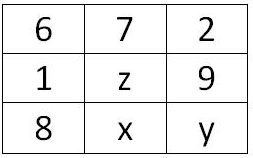Detailed Solution for RRB Group D Mock Test - 37 - Question 28

Each number is used only once and the sums of numbers along both the diagonals are equal.
6 + z + y = 2 + 8 + z
=>y = 4
6 + 1+ 8 = 6 + z + 4
=> z = 5 and x = 3.
(x,y,z) = (3,4,5).
Therefore, option A is the right answer.

RRB Group D Mock Test - 37 - Question 29

Consider the given statements to be true even if they are at odds with generally known things. Based on the statement, evaluate which of the conclusions follow:
Statements:

No tree is a herb.
All herbs are shrubs.
Some shrubs are trees.

Conclusions:
I: All shrubs being trees is a possibility.
II: All trees being shrubs is a possibility.

Detailed Solution for RRB Group D Mock Test - 37 - Question 29

The given statements can be expressed diagrammatically as follows without violating any of the conditions.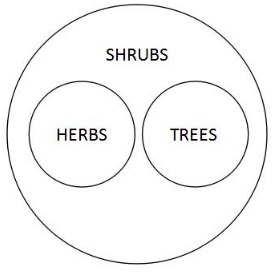Shrubs that are herbs cannot be trees as no herb is a tree. Therefore, conclusion I does not follow.
Trees and herbs can be 2 disjoint subsets of Shrubs. Therefore, conclusion II follows.

Only conclusion II follows. Therefore, option B is the right answer.

RRB Group D Mock Test - 37 - Question 30

Which of the following options will bear the same relationship as the words given in the first part of the question?
Belgium: glass :: Italy : ?

Detailed Solution for RRB Group D Mock Test - 37 - Question 30

Belgium is famous for glass products. Similarly, Italy is renowned for its marbles. Therefore, option C is the right answer.

RRB Group D Mock Test - 37 - Question 31

Which of the following options will bear the same relationship as the numbers given in the first part of the question?

371 : 4 :: 442 : ?

Detailed Solution for RRB Group D Mock Test - 37 - Question 31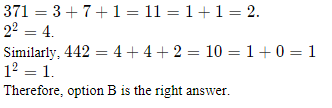RRB Group D Mock Test - 37 - Question 32

A family consists of 4 members - A, B, C and D. There are 2 male, 2 female members in the family. The family contains 2 married couples. C and A are related to each other by blood. A is related to B the same way D is related to C. If C is the father of A, how is D related to B?

Detailed Solution for RRB Group D Mock Test - 37 - Question 32

C and A are related to each other by blood. There are 2 married couples in the family. Therefore, the other 2 must be spouses of these 2 persons.

C is the father of A. A is related to B the same way D is related to C. Therefore, A must be the husband of B and D must be the husband of C. As we can see, D must be the son-in-law of B. Therefore, option A is the right answer.

RRB Group D Mock Test - 37 - Question 33

If 12 & 27 = 324 and 41 ! 73 = 114, then what is the value of 35 ! 16 & 18?

Detailed Solution for RRB Group D Mock Test - 37 - Question 33

35 ! 16 & 18 = 35 + 16*18 = 323
Hence, option C is the right answer.

RRB Group D Mock Test - 37 - Question 34

Which of the following Venn diagrams captures the relationship between predator, prey, goat, tiger, frog?

Detailed Solution for RRB Group D Mock Test - 37 - Question 34

Some animals are both predator and prey. Frog eats insects and snakes eat frog. Therefore, frog is both a predator and prey.

Goat is a prey. Goats are herbivores and hence, they can be purely termed as preys. Tiger is an apex predator.

Therefore, the Venn diagram must contain 2 large intersecting circles and 3 small circles, one in each of the regions formed by the intersecting circles. Only option C satisfies this condition. Hence, option C is the right answer.

RRB Group D Mock Test - 37 - Question 35

In the following diagram, each geometric shape represents a movie.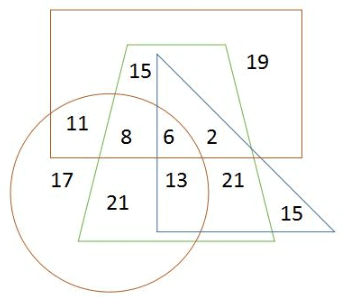The number of people who have watched exactly 3 movies is

Detailed Solution for RRB Group D Mock Test - 37 - Question 35

The region common to 3 shapes will give us the number of people who have watched exactly 3 movies.

From the diagram, the number of people who have watched exactly 3 movies is 8+2+13 = 23. Hence, option A is the right answer.

RRB Group D Mock Test - 37 - Question 36

Find the number which should replace ‘?’.
1, 9, 49, 169, ?

Detailed Solution for RRB Group D Mock Test - 37 - Question 36

The series is the squares of the first series:

The first series starts with 1 and subsequent terms are increased by 2, 4, 6, 8 ... and so on.
1
1 + 2 = 3
3 + 4 = 7
7 + 6 = 13
13 + 8 = 21

Squares of these numbers: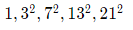RRB Group D Mock Test - 37 - Question 37

Ganesh walks 20 m towards the East. Then, he walks 3 m towards the south. He takes a turn and walks 4 m towards the East. Finally, he walks 10 m towards the North and comes to a halt. How far is Ganesh from his starting point?

Detailed Solution for RRB Group D Mock Test - 37 - Question 37

The given statements can be expressed diagrammatically as follows: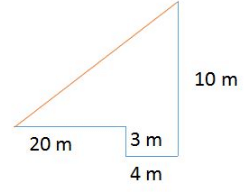Total distance travelled towards the East = 20 + 420+4 = 2424 m.
Total distance travelled towards the North = -3 + 10−3+10 = 77 m.
Distance from the starting point can be found using the Pythagoras theorem.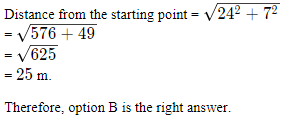RRB Group D Mock Test - 37 - Question 38

5 persons A, B, C, D and E live on 5 different floors of a building. The floors are numbered from 1 to 5, 1 being the topmost floor and 5 being the bottom most floor. A lives on an odd numbered floor above D. D has more persons living above him than below him. D lives on an even numbered floor. C lives on an odd numbered floor above D but below A. E lives on a floor above B. Who lives on floor 2?

Detailed Solution for RRB Group D Mock Test - 37 - Question 38

1 is the topmost floor and 5 is the bottom-most floor. A lives on an odd numbered floor above D. Therefore, A must be living on floor 1 or 3. D has more persons living above him than below him. Therefore, D must be living on floor 4 or 5. D lives on an even numbered floor. Therefore, D must be living on floor 4. C lives on an odd numbered floor above D but below A. Therefore, A must be living on floor 1 and C must be living on floor 3. E lives on a floor above B. Therefore, B must be living on floor 5 and E must be living on floor 2.

The final arrangement is as follows:

1 - A
2 - E
3 - C
4 - D
5 - B

As we can see, E lives on the second floor. Therefore, option B is the right answer.

RRB Group D Mock Test - 37 - Question 39

A machine codes DATE as 400 and TIME as 11700. The code for a 3 lettered word is 100. Which of the following cannot be the word?

Detailed Solution for RRB Group D Mock Test - 37 - Question 39

DATE is coded as 400.
DATE - 4*1*20*5 - 400
TIME - 20*9*13*5 - 11700

Let us evaluate the options one by one.
DAY - 4*1*25 - 100
TEA - 20*5*1 - 100
EAT - 5*1*20 - 100
BET - 2*5*20 - 200

Therefore, option D is the right answer.

RRB Group D Mock Test - 37 - Question 40

Find the mirror image of the word “banquet”.

Detailed Solution for RRB Group D Mock Test - 37 - Question 40

In option A, mirror image of 'n' and 'b' is incorrect.

In option B, mirror image of 'n' is incorrect.

In option C, mirror image of 'q' is incorrect.

Option D is the mirror image.

RRB Group D Mock Test - 37 - Question 41

To which sport is Leander Paes related to?

RRB Group D Mock Test - 37 - Question 42

On which of the following dates the President Pranab Mukherjee gave his assent to the Andhra Pradesh State Reorganization Bill to create Telangana as India’s 29th State?

RRB Group D Mock Test - 37 - Question 43

RRB Group D Mock Test - 37 - Question 44

CD-ROM stands for?

RRB Group D Mock Test - 37 - Question 45

Where is the headquarters of SAARC?

RRB Group D Mock Test - 37 - Question 46

Which of the following statements in incorrect

RRB Group D Mock Test - 37 - Question 47

In Boolean algebra (1 + 1).(0 + 0) = ?

RRB Group D Mock Test - 37 - Question 48

Which of the following includes meaningful interpretation of data and knowledge?

Detailed Solution for RRB Group D Mock Test - 37 - Question 48

Data are sensory stimuli that we perceive through our senses. Information is data that has been processed into a form that is meaningful to the recipient (Davis and Olson, 1985). Information is a set of significant signs that has the ability to create knowledge. The essence of the information phenomenon has been characterized as the occurrence of a communication process that takes place between the sender and the recipient of the message (Wersig and Neveling, 1975).

RRB Group D Mock Test - 37 - Question 49

The acronym "YAHOO" stands for:

RRB Group D Mock Test - 37 - Question 50

Rihand Dam is located in which state?

RRB Group D Mock Test - 37 - Question 51

Port Victoria is the capital of which country?

RRB Group D Mock Test - 37 - Question 52

In which part of the cell does gene mutation take place?

RRB Group D Mock Test - 37 - Question 53

What is the capital of South Korea?

RRB Group D Mock Test - 37 - Question 54

For stabilizing the gain of an amplifier

RRB Group D Mock Test - 37 - Question 55

Cardiology is the study of?

RRB Group D Mock Test - 37 - Question 56

A stereophonic system requires

RRB Group D Mock Test - 37 - Question 57

Garampani Sanctury is located in?

RRB Group D Mock Test - 37 - Question 58

Excess silica in cement

RRB Group D Mock Test - 37 - Question 59

The great Victoria Desert is located in

RRB Group D Mock Test - 37 - Question 60

The earth’s primary source of energy is

RRB Group D Mock Test - 37 - Question 61

Which famous independence leader gave the quote 'Jai Jawan, Jai Kisan'?

RRB Group D Mock Test - 37 - Question 62

Who is the author of book” Ashadhyani” on Sanskrit grammar?

RRB Group D Mock Test - 37 - Question 63

How many Major Ports are there in India?

RRB Group D Mock Test - 37 - Question 64

Which city is considered as "Manchester of India"?

RRB Group D Mock Test - 37 - Question 65

Which was the summer capital of India during the British Raj?

RRB Group D Mock Test - 37 - Question 66

Which of the following princely states supported the British during the Indian First War of Independence?

RRB Group D Mock Test - 37 - Question 67

Pulmonary Veins carry blood between which of the following Organs of the Body?

RRB Group D Mock Test - 37 - Question 68

The only female ruler of the Delhi Sultanate, Razia Sultana was the daughter of ….

RRB Group D Mock Test - 37 - Question 69

Which fuel has highest calorific value?

RRB Group D Mock Test - 37 - Question 70

Which Indian region is the only place in the world where the Asiatic Wild Ass is found?

RRB Group D Mock Test - 37 - Question 71

Raut Nacha is a folk dance of?

RRB Group D Mock Test - 37 - Question 72

Where is the headquarters of DRDO?

RRB Group D Mock Test - 37 - Question 73

hat is the chemical formula of Urea?

Detailed Solution for RRB Group D Mock Test - 37 - Question 73

The chemical formula of Urea is CH4​N2​O

RRB Group D Mock Test - 37 - Question 74

The respiratory gases in the human body is carried by which of the following cells?

RRB Group D Mock Test - 37 - Question 75

Which of the following is not a correct classification of Harmones based on their structure?

RRB Group D Mock Test - 37 - Question 76

Which of the following harmones secreted by the Thyroid Gland?

RRB Group D Mock Test - 37 - Question 77

The Hormone - Melatonin that helps in regulating the human Sleep- Wake Cycle is secreted by which of the following glands?

RRB Group D Mock Test - 37 - Question 78

The main chemical constituent of clay is

RRB Group D Mock Test - 37 - Question 79

Which of the following is on the left side of the photosynthesis equation?

RRB Group D Mock Test - 37 - Question 80

What is the unit of rate of heat transfer?

RRB Group D Mock Test - 37 - Question 81

Which leader’s birthday is celebrated annually as Teacher’s Day in India ?

RRB Group D Mock Test - 37 - Question 82

The wavelength of ultra-violet light is of which order?

RRB Group D Mock Test - 37 - Question 83

What is the chemical formula for laughing gas?Report Question

RRB Group D Mock Test - 37 - Question 84

Which of these are the main ingredients of LPG?

RRB Group D Mock Test - 37 - Question 85

What is the mathematical expression for the Newton's Second law of Motion?

Detailed Solution for RRB Group D Mock Test - 37 - Question 85

According to the Newton's second law of motion, F = ma. Here F is the force, m is the mass and a is the acceleration.

RRB Group D Mock Test - 37 - Question 86

Mary kom movie produced by Sanjay Leela Bhansali is written by?

RRB Group D Mock Test - 37 - Question 87

Who gave the principle of objects submerged partially or fully in water?

RRB Group D Mock Test - 37 - Question 88

What is the name of the Japanese space explorer that was launched on a 6-year round-trip journey to blow a crater in a remote asteroid and collect samples from inside in hope of gathering clues to the origin of the Earth?

RRB Group D Mock Test - 37 - Question 89

The ancient civilization of Sumer flourished in a region, which is a part of the following country in the modern world

RRB Group D Mock Test - 37 - Question 90

Who received the first Nobel Prize in Physics in India ?

RRB Group D Mock Test - 37 - Question 91

which among the following is the largest port in India?

RRB Group D Mock Test - 37 - Question 92

Which day is observed as the 'International Day of Older Persons' every year?

RRB Group D Mock Test - 37 - Question 93

Who is considered as the “Father of Genetics”?

RRB Group D Mock Test - 37 - Question 94

Who discovered the X-RAYS?

RRB Group D Mock Test - 37 - Question 95

Who invented electric battery?

RRB Group D Mock Test - 37 - Question 96

Which day is observed as the 'United Nations Formation Day'

RRB Group D Mock Test - 37 - Question 97

Who is the Deputy speaker of Loka sabha?

RRB Group D Mock Test - 37 - Question 98

Maneka Gandhi is the Union Minister for?

RRB Group D Mock Test - 37 - Question 99

Which of following countries meet more than one fourth of their energy requirement from nuclear power

RRB Group D Mock Test - 37 - Question 100

The national sport of which of these countries is Taekwondo?

## RRB (Group D) - Mock Tests & Previous Year Papers

1 docs|48 tests
 Use Code STAYHOME200 and get INR 200 additional OFF Use Coupon Code
Information about RRB Group D Mock Test - 37 Page
In this test you can find the Exam questions for RRB Group D Mock Test - 37 solved & explained in the simplest way possible. Besides giving Questions and answers for RRB Group D Mock Test - 37, EduRev gives you an ample number of Online tests for practice

1 docs|48 tests